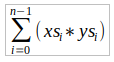# Solutions to the Problem Sheet for LI Functional Programming - Week 3

## 列表理解 List Comprehensions​

1. A triple (x,y,z) of positive integers is called pythagorean if $x^2 + y^2 = z^2$. Using a list comprehension, define a function that maps an integer n to all such triples with components in [1..n].

pyths :: Int -> [(Int,Int,Int)]pyths n = [ (a,b,c) | a <- ns , b <- ns , c <- ns , a^2 + b^2 == c^2 ]  where ns = [1..n]
2. A positive integer is perfect if it equals the sum of all of its factors, excluding the number itself. Using a list comprehension, define a function that returns the list of all perfect numbers up to a given limit. Many variations of this exercise are possible:

• A number which is less than the sum of its proper divisors is called abundant.
• A number which is greater than the sum of its proper divisions is called deficient.
• A number for which the sum of all its divisors (including itself) is greater than the sum of the divisors of any smaller number is called highly abundant.

For each of these variations, write a function which finds all the numbers with the stated property below a given number.

divisors :: Int -> [Int]divisors n = [ f | f <- [1 .. n-1] , n mod f == 0 ]perfects :: Int -> [Int]perfects n = [ p | p <- [1..n] , sum (divisors p) == p ]abundants :: Int -> [Int]abundants n = [ a | a <- [1..n] , sum (divisors a) > a ]deficients :: Int -> [Int]deficients n = [ a | a <- [1..n] , sum (divisors a) < a ]
highlyAbundant :: Int -> [Int]highlyAbundant n = [ a | a <- [1..n] ,                     and [ sum (a:divisors a) > sum (b:divisors b) | b <- [1..a-1] ] ]
1. The scalar product of two lists of integers xs and ys of length n is give by the sum of the products of the corresponding integers:Using a list comprehension, define a function that returns the scalar product of two lists.

dotProd :: [Int] -> [Int] -> IntdotProd x y = sum [ i * j | (i,j) <- zip x y ]
2. Harder Implement matrix multiplication where matricies are represented by lists of lists of integers. One possibility, for example, would be to take the dimensions of the matrices as arguments, so that your function would have type:

matrixMul :: Int -> Int -> Int -> [[Int]] -> [[Int]] -> [[Int]]matrixMul n p m x y =  [ [ sum [ ((x !! i) !! k) * ((y !! k) !! j) | k <- [0..p-1] ]    | j <- [0..m-1] ] | i <- [0..n-1] ]

## Recursive Functions​

1. Without looking at the standard prelude, define the following library functions using recursion:

• Decide if all logical values in a list are true:

and' :: [Bool] -> Booland' [] = Trueand' (x:xs) = x && (and' xs)
• Concatenate a list of lists:

concat' :: [[a]] -> [a]concat' [] = []concat' (x:xs) = x ++ concat' xs
• Produce a list with n identical elements:

replicate' :: Int -> a -> [a]replicate' n x | n <= 0 = []                 | otherwise = x : replicate' (n-1) x
• Select the nth element of a list:

nth :: [a] -> Int -> anth [] _ = undefinednth (x:xs) n | n == 0 = x               | otherwise = nth xs (n-1)
• Decide if a value is an element of a list:

elem' :: Eq a => a -> [a] -> Boolelem' y [] = Falseelem' y (x:xs) | x == y = True                 | otherwise = False
2. Define a recursive function that merges two sorted lists of values to give a single sorted list.

merge :: Ord a => [a] -> [a] -> [a]merge [] y = ymerge x [] = xmerge (x:xs) (y:ys) | x <= y = x:(merge xs (y:ys))                    | otherwise = y:(merge (x:xs) ys)

## Higher Order Functions​

1. What are higher-order functions that return functions as results better known as?

Just functions, or perhaps more specifically, curried functions.

2. Express the comprehension [f x | x <- xs, p x] using the functions map and filter.

fun :: Num a => (a -> a) -> (a -> Bool) -> [a] -> [a]fun f p xs = map f (filter p xs)
3. Redefine map f and filter p using foldr.

map' :: (a -> b) -> [a] -> [b]map' f xs = foldr (\x r -> (f x):r)  [] xsfilter' :: (a -> Bool) -> [a] -> [a]filter' p xs = foldr (\x r -> if p x then x:r else r) [] xs
4. Define a function altMap :: (a -> b) -> (a -> b) -> [a] -> [b] that alternatively applies the two argument functions to successive elements in a list.

altMap :: (a -> b) -> (a -> b) -> [a] -> [b]altMap f g [] = []altMap f g (x:[]) = f x : []altMap f g (x:y:xs) = (f x):(g y):(altMap f g xs)
5. Harder. Church Numerals

• Write a function to implement addition of Church numerals
• Write a function to implement multiplication of Church numerals
addChurch :: ((a -> a) -> (a -> a)) ->             ((a -> a) -> (a -> a)) ->             ((a -> a) -> (a -> a))addChurch m n f x = m f (n f x)mulChurch :: ((a -> a) -> (a -> a)) ->             ((a -> a) -> (a -> a)) ->             ((a -> a) -> (a -> a))mulChurch m n f = m (n f)`